# 4-Way 3-Position Directional Valve (IL)

4-way, 3-position directional valve in an isothermal liquid system

Since R2020a

•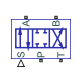Libraries:
Simscape / Fluids / Isothermal Liquid / Valves & Orifices / Directional Control Valves

## Description

The 4-Way 3-Position Directional Valve (IL) block models a valve with four openings in an isothermal liquid network, typically between an actuator, pump, and tank. The valve operation is controlled by a single spool displaced according to the signal at port S. You can set the baseline configuration of your valve by specifying the orifices that are open when the spool is in the neutral position (centered at 0 m) in the Neutral spool position open connections parameter, when the spool moves in the positive direction in the Positive spool position open connections parameter, and when the spool moves in the negative direction in the Negative spool position open connections.

You can set the model for valve opening in the Orifice parameterization parameter as a linear relationship or function of user-provided data, which can be applied to one or all flow paths in the valve.

Example Valve SetupIn this example configuration, Neutral spool position open connections is set to `All closed`. When the spool is in the neutral position, all orifices are closed to flow:In this configuration, Negative spool position open connections is set to `P-A and B-T`. When the signal at port S moves the spool to a negative position, the paths between ports P and A and between ports B and T are open to flow. The paths between ports P and B and ports A and T are closed to flow:In this configuration, Positive spool position open connections is set to `P-B and A-T`. When the signal at port S moves the spool to a positive position, the paths between ports P and B and between ports A and T are open to flow and the paths between ports P and A and between ports B and T are closed:Schematically, this configuration is shown as:The left-hand side corresponds to the positive position and the right-hand side corresponds to the negative position.

### Spool Displacement and Valve Configuration

The spool stroke is the amount of spool travel an orifice takes to fully open from a closed position and is defined for each orifice selected on the tab. The table below shows how the stroke is calculated in accordance with the valve orifice parameterization and flow path characteristics.

Spool Stroke Based on Parameterization and Flow Path

Orifice ParameterizationIdentical for All Flow PathsDifferent for Each Flow Path
Linear - Area vs. spool travelDefined by the Spool travel between closed and open orifice parameterDefined by the Spool travel between closed and open orifice parameter, for each orifice
Tabulated data - Area vs. spool travelThe difference between the first and last element of the Spool travel vector parameterThe difference between the first and last element of the spool travel vector parameter for each orifice
Tabulated data - Volumetric flow rate vs. spool travel and pressure dropThe difference between the first and last element of the Spool travel vector, ds parameterThe difference between the first and last element of the spool travel vector, ds parameter for each orifice

The section Visualize Orifice Openings details how to see the orientation of all valve orifices.

The direction of spool movement signaled at port S depends on the spool stroke and the orifice orientation:

Orifice Definition and Spool Travel Direction

Spool Position when Orifice is OpenSpool Travel, ΔS
Always closed (orifice is not selected in any spool position configuration)N/A
Always open (orifice is selected in positive, neutral, and negative spool position configurations)N/A
NegativeSorifice_max + spool stroke – S
Negative and NeutralSorifice_max + spool stroke – S
PositiveSorifice_max – spool stroke + S
Positive and NeutralSorifice_max – spool stroke + S
Neutral|Sorifice_max| + spool stroke – |S|
Positive and Negative–|Sorifice_max| + spool stroke – |S|

Where Sorifice_max is the spool position at the maximum orifice area defined for each orifice. The orifice area saturates at the leakage area when ΔS is negative and saturates at the maximum orifice area when the orifice is fully open. When the orifice is fully open, ΔS is equal to the spool stroke, so ΔS greater than the spool stroke does not further increase the orifice area.

The Consistency check for neutral spool position open connections parameter verifies that

• Any orifice listed in Neutral spool position open connections is open when the signal at S is 0.

• Any orifice that is not listed in Neutral spool position open connections is closed when the signal at S is 0.

You can optionally choose to receive a warning or error when this check is violated.

The possible configurations of the 4-Way 3-Position Directional Valve (IL) block are shown below.

Note

This block includes all configurations from the Hydraulic (Isothermal) library blocks 4-Way Directional Valve and the 4-Way Directional Valves A – K.

4-Way 3-Position Directional Valve Configuration

ConfigurationParameter Values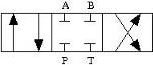All four orifices are closed in the neutral position. (default)

• Positive spool position open connections: ```P-A and B-T```

• Neutral spool position open connections: ```All closed```

• Negative spool position open connections: ```P-B and A-T```

• Spool position at maximum P-A orifice area > P-A spool stroke

• Spool position at maximum P-B orifice area < –P-B spool stroke

• Spool position at maximum A-T orifice area < –A-T spool stroke

• Spool position at maximum B-T orifice area > B-T spool strokeAll four orifices are open in the neutral position.

• Positive spool position open connections: ```P-A and B-T```

• Neutral spool position open connections: ```P-A, P-B, A-T, and B-T```

• Negative spool position open connections: ```P-B and A-T```

• Spool position at maximum P-A orifice area < P-A spool stroke

• Spool position at maximum P-B orifice area > –P-B spool stroke

• Spool position at maximum A-T orifice area > –A-T spool stroke

• Spool position at maximum B-T orifice area < B-T spool stroke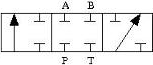Orifices A-T and B-T are closed. Orifices P-A and P-B are overlapped.

• Positive spool position open connections: ```P-A only```

• Neutral spool position open connections: ```All closed```

• Negative spool position open connections: ```P-B only```

• Spool position at maximum P-A orifice area > P-A spool stroke

• Spool position at maximum P-B orifice area < –P-B spool stroke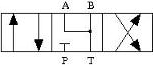Orifices A-T and B-T are open. Orifices P-A and P-B are overlapped.

• Positive spool position open connections: ```P-A and B-T```

• Neutral spool position open connections: ```A-T and B-T```

• Negative spool position open connections: ```P-B and A-T```

• Spool position at maximum P-A orifice area > P-A spool stroke

• Spool position at maximum P-B orifice area < –P-B spool stroke

• Spool position at maximum A-T orifice area > –A-T spool stroke

• Spool position at maximum B-T orifice area < B-T spool stroke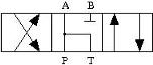Orifices P-A and A-T are open in the neutral position. Orifices P-B and B-T are overlapped.

• Positive spool position open connections: ```P-B and A-T```

• Neutral spool position open connections: ```P-A and A-T```

• Negative spool position open connections: ```P-A and B-T```

• Spool position at maximum P-A orifice area < P-A spool stroke

• Spool position at maximum P-B orifice area > P-B spool stroke

• Spool position at maximum A-T orifice area > A-T spool stroke

• Spool position at maximum B-T orifice area < –B-T spool stroke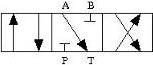Orifice A-T is open in the neutral position. Orifices P-B, A-T, and B-T are overlapped.

• Positive spool position open connections: ```P-A and B-T```

• Neutral spool position open connections: ```A-T only```

• Negative spool position open connections: ```P-B and A-T```

• Spool position at maximum P-A orifice area > P-A spool stroke

• Spool position at maximum P-B orifice area < –P-B spool stroke

• Spool position at maximum A-T orifice area > –A-T spool stroke

• Spool position at maximum B-T orifice area > B-T spool stroke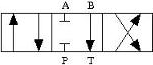Orifice B-T is open in the neutral position. Orifices P-A, P-B, and A-T are overlapped.

• Positive spool position open connections: ```P-A and B-T```

• Neutral spool position open connections: ```B-T only```

• Negative spool position open connections: ```P-B and A-T```

• Spool position at maximum P-A orifice area > P-A spool stroke

• Spool position at maximum P-B orifice area < –P-B spool stroke

• Spool position at maximum A-T orifice area < –A-T spool stroke

• Spool position at maximum B-T orifice area < B-T spool stroke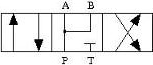Orifices P-A and P-B are open. Orifices A-T and B-T are overlapped.

• Positive spool position open connections: ```P-A and B-T```

• Neutral spool position open connections: ```P-A and P-B```

• Negative spool position open connections: ```P-B and A-T```

• Spool position at maximum P-A orifice area < P-A spool stroke

• Spool position at maximum P-B orifice area > –P-B spool stroke

• Spool position at maximum A-T orifice area < –A-T spool stroke

• Spool position at maximum B-T orifice area > B-T spool stroke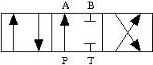Orifice P-A is open in the neutral position. Orifices P-B, A-T, and B-T are overlapped.

• Positive spool position open connections: ```P-A and B-T```

• Neutral spool position open connections: ```P-A only```

• Negative spool position open connections: ```P-B and A-T```

• Spool position at maximum P-A orifice area < P-A spool stroke

• Spool position at maximum P-B orifice area < –P-B spool stroke

• Spool position at maximum A-T orifice area < –A-T spool stroke

• Spool position at maximum B-T orifice area > B-T spool stroke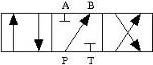Orifice P-B is open in the neutral position. Orifices P-A, A-T, and B-T are overlapped.

• Positive spool position open connections: ```P-A and B-T```

• Neutral spool position open connections: ```P-B only```

• Negative spool position open connections: ```P-B and A-T```

• Spool position at maximum P-A orifice area > P-A spool stroke

• Spool position at maximum P-B orifice area > –P-B spool stroke

• Spool position at maximum A-T orifice area < –A-T spool stroke

• Spool position at maximum B-T orifice area > B-T spool stroke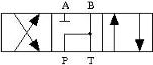Orifices P-B and B-T are open in the neutral position. Orifices P-A and A-T are overlapped.

• Positive spool position open connections: ```P-B and A-T```

• Neutral spool position open connections: ```P-B and B-T```

• Negative spool position open connections: ```P-A and B-T```

• Spool position at maximum P-A orifice area < –P-A spool stroke

• Spool position at maximum P-B orifice area < P-B spool stroke

• Spool position at maximum A-T orifice area > A-T spool stroke

• Spool position at maximum B-T orifice area > –B-T spool stroke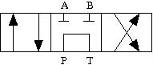Orifices P-A, P-B, A-T, and B-T are closed in the neutral position. P-T is open in the neutral position and closes when the spool displaces.

• Positive spool position open connections: ```P-A and B-T```

• Neutral spool position open connections: ```P-T only```

• Negative spool position open connections: ```P-B and A-T```

• Spool position at maximum P-A orifice area > P-A spool stroke

• Spool position at maximum P-B orifice area < –P-B spool stroke

• Spool position at maximum A-T orifice area < –A-T spool stroke

• Spool position at maximum B-T orifice area > B-T spool stroke

• Spool position at maximum P-T orifice area = any value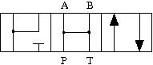Orifice P-A is a constant orifice. Orifices P-B, A-T, and B-T are open in the neutral position.

• Positive spool position open connections: ```P-A and P-B```

• Neutral spool position open connections: ```P-A, P-B, A-T, and B-T```

• Negative spool position open connections: ```P-A and B-T```

• Spool position at maximum P-A orifice area < P-A spool stroke

• Spool position at maximum P-B orifice area < P-B spool stroke

• Spool position at maximum A-T orifice area = any value

• Spool position at maximum B-T orifice area > –B-T spool stroke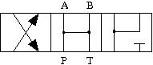Orifice P-B is a constant orifice. Orifices P-A, A-T and B-T are open in the neutral position.

• Positive spool position open connections: ```P-B and A-T```

• Neutral spool position open connections: ```P-A, P-B, A-T, and B-T```

• Negative spool position open connections: ```P-A and P-B```

• Spool position at maximum P-A orifice area > –P-A spool stroke

• Spool position at maximum A-T orifice area < A-T spool stroke

• Spool position at maximum B-T orifice area = any value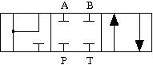Orifice P-A is closed in the neutral position. Ports A and T do not form a flow path.

• Positive spool position open connections: ```P-A and P-B```

• Neutral spool position open connections: ```All closed```

• Negative spool position open connections: ```P-A and B-T```

• |Spool position at maximum P-A orifice area| > P-A spool stroke

• Spool position at maximum P-B orifice area > P-B spool stroke

• Spool position at maximum B-T orifice area < –B-T spool stroke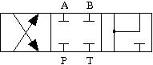Orifice P-B is closed in the neutral position. Ports B and T do not form a flow path.

• Positive spool position open connections: ```P-B and A-T```

• Neutral spool position open connections: ```All closed```

• Negative spool position open connections: ```P-A and P-B```

• Spool position at maximum P-A orifice area < –P-A spool stroke

• |Spool position at maximum P-B orifice area| > P-B spool stroke

• Spool position at maximum A-T orifice area > A-T spool stroke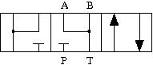Orifice P-A is closed in the neutral position. Orifice A-T is open in the neutral position.

• Positive spool position open connections: ```P-A and P-B```

• Neutral spool position open connections: ```A-T and B-T```

• Negative spool position open connections: ```P-A and B-T```

• |Spool position at maximum P-A orifice area| > P-A spool stroke

• Spool position at maximum P-B orifice area > P-B spool stroke

• Spool position at maximum A-T orifice area = any value

• Spool position at maximum B-T orifice area > –B-T spool strokeOrifice P-B is closed in the neutral position. Orifice B-T is open in the neutral position.

• Positive spool position open connections: ```P-B and A-T```

• Neutral spool position open connections: ```A-T and B-T```

• Negative spool position open connections: ```P-A and P-B```

• Spool position at maximum P-A orifice area < –P-A spool stroke

• |Spool position at maximum P-B orifice area| > P-B spool stroke

• Spool position at maximum A-T orifice area < A-T spool stroke

• Spool position at maximum B-T orifice area = any value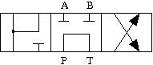Orifice P-B is closed in the neutral position. Orifice P-T is open in the neutral position. Ports A and T do not form a flow path.

• Positive spool position open connections: ```P-A and P-B```

• Neutral spool position open connections: ```P-T only```

• Negative spool position open connections: ```P-B and A-T```

• Spool position at maximum P-A orifice area > P-A spool stroke

• |Spool position at maximum P-B orifice area| > P-B spool stroke

• Spool position at maximum B-T orifice area < –B-T spool stroke

• Spool position at maximum P-T orifice area = any value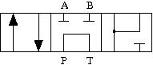Orifice P-A is closed in the neutral position. Orifice P-T is open in the neutral position. Ports A and T do not form a flow path.

• Positive spool position open connections: ```P-A and B-T```

• Neutral spool position open connections: ```P-T only```

• Negative spool position open connections: ```P-A and P-B```

• |Spool position at maximum P-A orifice area| > P-A spool stroke

• Spool position at maximum P-B orifice area < –P-B spool stroke

• Spool position at maximum B-T orifice area > B-T spool stroke

• Spool position at maximum P-T orifice area = any value

### Valve Orifice Parameterizations

The Orifice parameterization parameter sets the model for the open area or volumetric flow rate through one or all of the valve orifices. The block applies the same data for all flow paths if Area characteristics is set to ```Identical for all flow paths```; otherwise, individual parameterizations are applied for the `Different for all flow paths` setting. You can model valve opening in three ways:

• `Linear - area vs. spool travel`

The opening area is a linear function of the spool position received as a signal at port S:

`${A}_{orifice}=\frac{\left({A}_{\mathrm{max}}-{A}_{leak}\right)}{\Delta {S}_{\mathrm{max}}}\Delta S+{A}_{leak},$`

where:

Aorifice saturates at Aleak when ΔS is negative and saturates at Amax when the orifice is fully open. When the orifice is fully open, ΔS is equal to the spool stroke, so ΔS greater than the spool stroke does not further increase the orifice area.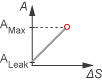When the valve is in a near-open or near-closed position in the linear parameterization, you can maintain numerical robustness in your simulation by adjusting the parameter. If the parameter is nonzero, the block smoothly saturates the opening area between Aleak and Amax. For more information, see Numerical Smoothing.

• `Tabulated data - Area vs. spool travel`

Provide spool travel vectors for your system or for individual flow paths between ports P, A, B, and T. This data will be used to calculate the relationship between the orifice area and spool displacement. Interpolation is used to determine the opening area between given data points. Aleak and Amax are the first and last parameters of the opening area vector, respectively.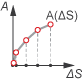• ```Tabulated data - Volumetric flow rate vs. spool travel and pressure drop```

Provide spool travel and pressure drop vectors and a dependent, 2-D volumetric flow rate array. Interpolation is used to determine flow rate between given data points. The mass flow rate is the product of the volumetric flow rate and the local density.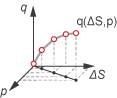### Visualize Orifice Openings

To visualize spool offsets and maximum displacement, right-click the block and select Fluids > Plot Valve Characteristics. The plot shows the orifices selected in the settings. The orifice parameterization sets the axes, which are either:

• Orifice area versus spool position

• Volumetric flow rate versus spool position, queried at a specific pressure differential

When the positive, neutral, and negative settings in the feature the same orifice, the plot displays the orifice openings as a constant.

To update the data after changing the block parameters, click on the figure window.

This image shows an example valve configuration. In the settings:

• Positive spool position open connections is `P-A and B-T`.

• Neutral spool position open connections is `P-T only`.

• Negative spool position open connections is `P-B and A-T`.

In the P-A Orifice settings:

• Spool position at maximum P-A orifice area is `2.5e-3 m`.

All other spool positions are at the default values.

Example Spool Position Visualization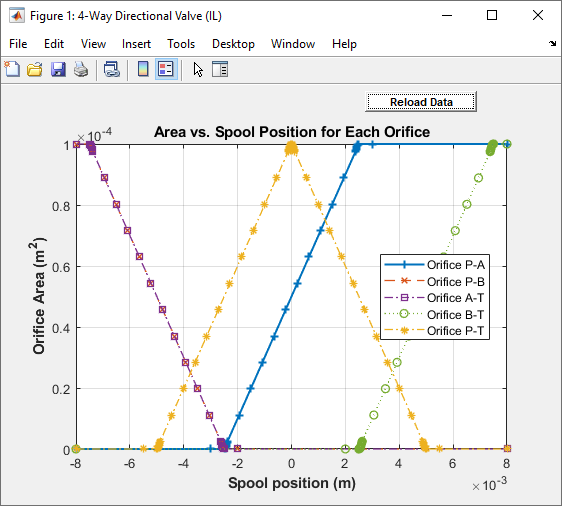The visualization shows that the P-A orifice is open between the spool position at `-2.53e-3` and `2.53e-3` m. Because the P-A orifice is open only when the spool is displaced in the positive direction, setting the Consistency check for neutral spool and position open connections parameter to `Warning` returns:

```Warning: P-A orifice must be closed when the spool is in the neutral position. Adjust Spool position at maximum P-A orifice area, Neutral spool position open connections, or Consistency check for neutral spool position open connections. Right-click on the block and select Fluids > Plot Valve Characteristics to view the area or flow of each connection versus spool position.```

To resolve this warning, you can change:

• Spool position at maximum P-A orifice area to a value larger than 0.005 m.

• Consistency check for neutral spool position open connections to `None`.

• The valve configuration by setting Neutral spool position open connections to ```P-A only```.

### Faults

When faults are enabled, the valve open area becomes stuck at a specified spool position in response to one of these triggers:

• Simulation time — Faulting occurs at a specified time.

• Simulation behavior — Faulting occurs in response to an external trigger. This exposes port Tr.

Four fault options are available in the Spool position when faulted parameter:

• `Positive` — The spool position freezes in the positive position, opening the Positive spool position open connections flow paths to their maximum value. The flow paths that are only open in the Negative spool position open connections and Neutral spool position open connections are closed.

• `Neutral` — The spool position freezes in the neutral position, opening all flow paths to their specified values at 0 m.

• `Negative` — The spool position freezes in the negative position, opening the Negative spool position open connections flow paths to their maximum value. The flow paths that are only open in the Positive spool position open connections and Neutral spool position open connections are closed.

• `Maintain last value` — The valve freezes at the spool position when the trigger occurs.

Due to numerical smoothing at the extremes of the valve area, in the linear parameterization, the minimum area applied is larger than the , and the maximum is smaller than the Maximum orifice area, in proportion to the Smoothing factor value.

Once triggered, the valve remains at the faulted area for the rest of the simulation.

### Composite Structure

This block is a composite component comprising of multiple instances of the Orifice (IL) block. Refer to the Orifice (IL) block for more detail on the valve parameterizations and block calculations.

### Predefined Parameterization

You can populate the block with pre-parameterized manufacturing data, which allows you to model a specific supplier component.

1. In the block dialog box, next to Selected part, click the "<click to select>" hyperlink next to Selected part in the block dialogue box settings.

2. The Block Parameterization Manager window opens. Select a part from the menu and click Apply all. You can narrow the choices using the Manufacturer drop down menu.

3. You can close the Block Parameterization Manager menu. The block now has the parameterization that you specified.

4. You can compare current parameter settings with a specific supplier component in the Block Parameterization Manager window by selecting a part and viewing the data in the Compare selected part with block section.

Note

Predefined block parameterizations use available data sources to supply parameter values. The block substitutes engineering judgement and simplifying assumptions for missing data. As a result, expect some deviation between simulated and actual physical behavior. To ensure accuracy, validate the simulated behavior against experimental data and refine your component models as necessary.

## Ports

### Conserving

expand all

Liquid entry or exit port to the valve.

Liquid entry or exit port to the valve.

Liquid entry or exit port to the valve.

Liquid entry or exit port to the valve.

### Input

expand all

Spool displacement, in m, specified as a physical signal.

Physical signal port for an external fault trigger. Triggering occurs when the value is greater than 0.5. There is no unit associated with the trigger value.

#### Dependencies

This port is visible when Enable faults is set to `On` and Fault trigger is set to `External`.

## Parameters

expand all

### Valve Configuration

Flow paths open when the spool is in a positive position. This parameter sets the valve configuration and defines the direction of spool movement according to the signal received at port S. The settings for this parameter can be checked against the definitions for the other orifices for consistency when the Consistency check for neutral spool position open connections is set to `Warning` or `Error`.

Flow paths open when the spool is in the neutral position. This parameter sets the valve configuration and defines the direction of spool movement according to the signal received at port S. The settings for this parameter can be checked against the definitions for the other orifices for consistency when the Consistency check for neutral spool position open connections is set to `Warning` or `Error`.

Flow paths open when the spool moves in the negative direction. This parameter sets the valve configuration and defines the direction of spool movement according to the signal received at port S. The settings for this parameter can be checked against the definitions for the other orifices for consistency when the Consistency check for neutral spool position open connections is set to `Warning` or `Error`.

Whether to check if the neutral spool position open settings on the Valve Configuration tab are consistent with the specified spool position at the maximum orifice area. For each enabled orifice, the actual offset at the spool neutral position should be commensurate with the specified spool offset for each individual orifice.

### Model Parameterization

Applies uniform or individual flow equations for the valve orifice area. `Identical for all flow paths` uses the same orifice and spool geometries, flow rates, pressure, and area vectors for all valve orifices. When using ```Different for each flow path```, these parameters are specified individually for each orifice. In both cases, orifices A, B, P, and T have the same cross-sectional areas, discharge coefficients, and Reynolds numbers in the `Linear - Area vs. spool travel` and `Tabulated data - Area vs. spool travel` parameterizations. The same Orifice parameterization is applied to all flow paths.

Method of calculating the valve opening area. In the tabulated data parameterizations, you can provide your own valve area and spool travel data for nonlinear valve opening profiles, or you can provide data in terms of volumetric flow rate, spool travel, and pressure drop over the flow path.

Maximum distance of spool travel.

#### Dependencies

To enable this parameter, set Area characteristics to ```Identical for all flow paths``` and Orifice parameterization to ```Linear - Area vs. spool travel```.

Largest open area of each orifice during the operation of the valve.

#### Dependencies

To enable this parameter, set Area characteristics to ```Identical for all flow paths``` and Orifice parameterization to ```Linear - Area vs. spool travel```.

Open area when an orifice is fully closed. Any area smaller than this value is maintained at the specified leakage area. This parameter contributes to numerical stability by maintaining continuity in the flow.

#### Dependencies

To enable this parameter, set Orifice parameterization to ```Linear - Area vs. spool travel```.

Vector of spool travel distances for the tabular parameterization of valve area. The vector elements must correspond one-to-one with the elements in the Orifice area vector parameter. The values are listed in ascending order and the first element must be 0. Linear interpolation is employed between table data points.

#### Dependencies

To enable this parameter, set Area characteristics to ```Identical for all flow paths``` and Orifice parameterization to ```Tabulated data - Area vs. spool travel```.

Vector of opening areas for the tabular parameterization of valve opening area. The vector elements must correspond one-to-one with the elements in the Spool travel vector parameter. The elements are listed in ascending order and must be greater than 0.

#### Dependencies

To enable this parameter, set Area characteristics to ```Identical for all flow paths``` and Orifice parameterization to ```Tabulated data - Area vs. spool travel```.

Vector of spool travel distances for the tabular parametrization of volumetric flow rate. The spool travel vector forms an independent axis with the Pressure drop vector, dp parameter for the 2-D dependent Volumetric flow rate table, q(ds,dp) parameter. The values are listed in ascending order and the first element must be 0. Linear interpolation is employed between table data points.

#### Dependencies

To enable this parameter, set Area characteristics to ```Identical for all flow paths``` and Orifice parameterization to ```Tabulated data - Volumetric flow rate vs. spool travel and pressure drop```.

Vector of pressure drop values for the tabular parametrization of volumetric flow rate. The pressure drop vector forms an independent axis with the Spool travel vector, ds parameter for the 2-D dependent Volumetric flow rate table, q(ds,dp) parameter. The values are listed in ascending order and must be greater than 0. Linear interpolation is employed between table data points.

#### Dependencies

To enable this parameter, set Area characteristics to ```Identical for all flow paths``` and Orifice parameterization to ```Tabulated data - Volumetric flow rate vs. spool travel and pressure drop```.

M-by-N matrix of volumetric flow rates based on independent values of pressure drop and spool travel distance. M and N are the sizes of the correlated vectors:

• M is the number of vector elements in the parameter.

• N is the number of vector elements in the Pressure drop vector, dp parameter.

#### Dependencies

To enable this parameter, set Area characteristics to ```Identical for all flow paths``` and Orifice parameterization to ```Tabulated data - Volumetric flow rate vs. spool travel and pressure drop```.

Orifice area when the positive, neutral, and negative spool positions feature the same orifice.

#### Dependencies

To enable this parameter, set Area characteristics to ```Identical for all flow paths``` and Orifice parameterization to either:

• ```Linear - area vs. spool travel```.

• ```Tabulated data - Area vs. spool travel```

The same orifice must be selected in all three of the Valve Configuration parameters:

• Positive spool position open connections

• Neutral spool position open connections

• Negative spool position open connections

Vector of pressure differential values over the valve when the positive, neutral, and negative spool positions feature the same orifice. The vector elements correspond one-to-one with the elements in the Constant orifice volumetric flow rate vector parameter. The values are listed in ascending order. Linear interpolation is employed between table data points.

#### Dependencies

To enable this parameter, set Area characteristics to ```Identical for all flow paths``` and Orifice parameterization to ```Tabulated data - Volumetric flow rate vs. spool travel and pressure drop```.

The same orifice must be selected in all three of the Valve Configuration parameters:

• Positive spool position open connections

• Neutral spool position open connections

• Negative spool position open connections

Vector of volumetric flow rate values for a constant orifice when the positive, neutral, and negative spool positions feature the same orifice. The vector elements correspond one-to-one with the elements in the Constant orifice pressure drop vector parameter. The values are listed in ascending order. Linear interpolation is employed between table data points.

#### Dependencies

To enable this parameter, set Area characteristics to ```Identical for all flow paths``` and Orifice parameterization to ```Tabulated data - Volumetric flow rate vs. spool travel and pressure drop```.

The same orifice must be selected in all three of the Valve Configuration parameters:

• Positive spool position open connections

• Neutral spool position open connections

• Negative spool position open connections

Maximum cross-sectional areas at the entry and exit ports P, T, A, and B, which are used in the pressure-flow rate equation that determines the mass flow rate through the valve.

#### Dependencies

To enable this parameter, set Area characteristics to ```Identical for all flow paths``` and Orifice parameterization to either:

• ```Linear - area vs. spool travel```

• ```Tabulated data - Area vs. spool travel```

Correction factor that accounts for discharge losses in theoretical flows.

#### Dependencies

To enable this parameter, set Orifice parameterization to either:

• ```Linear - Area vs. spool travel```

• ```Tabulated data - Area vs. spool travel```

Upper Reynolds number limit for laminar flow through the orifice.

#### Dependencies

To enable this parameter, set Orifice parameterization to:

• ```Linear - area vs. spool travel```

• ```Tabulated data - Area vs. spool travel```

Continuous smoothing factor that introduces a layer of gradual change based to the flow response when the valve is in near-open and near-closed positions. To increase the stability of your simulation in these regimes, set this parameter to a nonzero value less than one.

#### Dependencies

To enable this parameter, set Orifice parametrization to ```Linear - area vs. spool travel```.

Whether to account for pressure increase when fluid flows from a region of smaller cross-sectional area to a region of larger cross-sectional area.

#### Dependencies

To enable this parameter, set Orifice parameterization to:

• ```Linear - area vs. spool travel```

• ```Tabulated data - Area vs. spool travel```

### P-A Orifice

Spool offset from the neutral position for a fully open P-A orifice. The Consistency check for neutral spool position open connections parameter will notify you if the values set for this spool position are inconsistent or unphysical according to other orifice spool positions. To visualize the defined offsets, see Visualize Orifice Openings.

#### Dependencies

To enable this parameter, set one of the following parameters:

Positive spool position open connections to:

• `P-A and B-T`

• `P-A and P-B`

• `P-A only`

Neutral spool position open connections to:

• ```P-A, P-B, A-T, and B-T```

• `P-A and A-T`

• `P-A and B-T`

• `P-A and P-B`

• `P-A only`

Negative spool position open connections to:

• ```P-A, P-B, A-T, and B-T```

• `P-A and A-T`

• `P-A and B-T`

• `P-A and P-B`

• `P-A only`

Note:

This parameter is enabled only when the P-A orifice settings do not appear in all three spool position parameters: Positive spool position open connections, Neutral spool position open connections, and Negative spool position open connections.

Maximum distance of spool travel. This value provides an upper limit to calculations so that simulations do not return unphysical values.

#### Dependencies

To enable this parameter, set:

1. Area characteristics to ```Different for each flow path```.

2. Orifice parameterization to ```Linear - Area vs. spool travel```.

3. One of the following parameters:

Positive spool position open connections to:

• ```P-A and B-T```

• ```P-A and P-B```

• `P-A only`

Neutral spool position open connections to:

• ```P-A, P-B, A-T, and B-T```

• ```P-A and A-T```

• ```P-A and B-T```

• ```P-A and P-B```

• `P-A only`

Negative spool position open connections to:

• ```P-A, P-B, A-T, and B-T```

• ```P-A and A-T```

• ```P-A and B-T```

• ```P-A and P-B```

• `P-A only`

Largest open area of the P-A orifice during the operation of the valve.

#### Dependencies

To enable this parameter, set:

1. Area characteristics to ```Different for each flow path```.

2. Orifice parameterization to ```Linear - Area vs. spool travel```.

3. One of the following parameters:

Positive spool position open connections to:

• ```P-A and B-T```

• ```P-A and P-B```

• `P-A only`

Neutral spool position open connections to:

• ```P-A, P-B, A-T, and B-T```

• ```P-A and A-T```

• ```P-A and B-T```

• ```P-A and P-B```

• `P-A only`

Negative spool position open connections to:

• ```P-A, P-B, A-T, and B-T```

• ```P-A and A-T```

• ```P-A and B-T```

• ```P-A and P-B```

• `P-A only`

Vector of spool travel distances for the tabular parameterization of valve area. The vector elements must correspond one-to-one with the elements in the P-A orifice area vector parameter. The values are listed in ascending order and the first element must be 0. Linear interpolation is employed between table data points.

#### Dependencies

To enable this parameter, set:

1. Area characteristics to ```Different for each flow path```.

2. Orifice parameterization to ```Tabulated data - Area vs. spool travel```.

3. One of the following parameters:

Positive spool position open connections to:

• ```P-A and B-T```

• ```P-A and P-B```

• `P-A only`

Neutral spool position open connections to:

• ```P-A, P-B, A-T, and B-T```

• ```P-A and A-T```

• ```P-A and B-T```

• ```P-A and P-B```

• `P-A only`

Negative spool position open connections to:

• ```P-A, P-B, A-T, and B-T```

• ```P-A and A-T```

• ```P-A and B-T```

• ```P-A and P-B```

• `P-A only`

Vector of opening areas for the tabular parameterization of valve opening area. The vector elements must correspond one-to-one with the elements in the P-A spool travel vector parameter. The elements are listed in ascending order and must be greater than 0.

#### Dependencies

To enable this parameter, set:

1. Area characteristics to ```Different for each flow path```.

2. Orifice parameterization to ```Tabulated data - Area vs. spool travel```.

3. One of the following parameters:

Positive spool position open connections to:

• ```P-A and B-T```

• ```P-A and P-B```

• `P-A only`

Neutral spool position open connections to:

• ```P-A, P-B, A-T, and B-T```

• ```P-A and A-T```

• ```P-A and B-T```

• ```P-A and P-B```

• `P-A only`

Negative spool position open connections to:

• ```P-A, P-B, A-T, and B-T```

• ```P-A and A-T```

• ```P-A and B-T```

• ```P-A and P-B```

• `P-A only`

Vector of spool travel distances for the tabular parameterization of volumetric flow rates. The spool travel vector forms an independent axis with the P-A orifice pressure drop vector, dp parameter for the 2-D dependent P-A orifice volumetric flow rate table, q(ds,dp) parameter. The values are listed in ascending order and the first element must be 0. Linear interpolation is employed between table data points.

#### Dependencies

To enable this parameter, set:

1. Area characteristics to ```Different for each flow path```.

2. Orifice parameterization to ```Tabulated data - Volumetric flow rate vs. spool travel and pressure drop```.

3. One of the following parameters:

Positive spool position open connections to:

• ```P-A and B-T```

• ```P-A and P-B```

• `P-A only`

Neutral spool position open connections to:

• ```P-A, P-B, A-T, and B-T```

• ```P-A and A-T```

• ```P-A and B-T```

• ```P-A and P-B```

• `P-A only`

Negative spool position open connections to:

• ```P-A, P-B, A-T, and B-T```

• ```P-A and A-T```

• ```P-A and B-T```

• ```P-A and P-B```

• `P-A only`

Vector of pressure drop values for the tabular parametrization of volumetric flow rate. The pressure drop vector forms an independent axis with the P-A orifice spool travel vector, ds parameter for the 2-D dependent P-A orifice volumetric flow rate table, q(ds,dp) parameter. The values are listed in ascending order and must be greater than 0. Linear interpolation is employed between table data points.

#### Dependencies

To enable this parameter, set:

1. Area characteristics to ```Different for each flow path```.

2. Orifice parameterization to ```Tabulated data - Volumetric flow rate vs. spool travel and pressure drop```.

3. One of the following parameters:

Positive spool position open connections to:

• ```P-A and B-T```

• ```P-A and P-B```

• `P-A only`

Neutral spool position open connections to:

• ```P-A, P-B, A-T, and B-T```

• ```P-A and A-T```

• ```P-A and B-T```

• ```P-A and P-B```

• `P-A only`

Negative spool position open connections to:

• ```P-A, P-B, A-T, and B-T```

• ```P-A and A-T```

• ```P-A and B-T```

• ```P-A and P-B```

• `P-A only`

M-by-N matrix of volumetric flow rates based on independent values of pressure drop and spool travel distance. M and N are the sizes of the correlated vectors:

• M is the number of vector elements in the parameter.

• N is the number of vector elements in the P-A orifice pressure drop vector, dp parameter.

#### Dependencies

To enable this parameter, set:

1. Area characteristics to ```Different for each flow path```.

2. Orifice parameterization to ```Tabulated data - Volumetric flow rate vs. spool travel and pressure drop```.

3. One of the following parameters:

Positive spool position open connections to:

• ```P-A and B-T```

• ```P-A and P-B```

• `P-A only`

Neutral spool position open connections to:

• ```P-A, P-B, A-T, and B-T```

• ```P-A and A-T```

• ```P-A and B-T```

• ```P-A and P-B```

• `P-A only`

Negative spool position open connections to:

• ```P-A, P-B, A-T, and B-T```

• ```P-A and A-T```

• ```P-A and B-T```

• ```P-A and P-B```

• `P-A only`

Orifice area when the positive, neutral, and negative spool positions feature the same orifice.

#### Dependencies

To enable this parameter, set:

1. Area characteristics to ```Different for each flow path```.

2. Orifice parameterization to either:

• ```Linear - area vs. spool travel```

• ```Tabulated data - Area vs. spool travel```

3. One of the following settings in each parameter:

Positive spool position open connections to:

• ```P-A and B-T```

• ```P-A and P-B```

• `P-A only`

Neutral spool position open connections to:

• ```P-A, P-B, A-T, and B-T```

• ```P-A and A-T```

• ```P-A and B-T```

• ```P-A and P-B```

• `P-A only`

Negative spool position open connections to:

• ```P-A, P-B, A-T, and B-T```

• ```P-A and A-T```

• ```P-A and B-T```

• ```P-A and P-B```

• `P-A only`

Vector of pressure differential values over the valve when the positive, neutral, and negative spool positions feature the same orifice. The vector elements correspond one-to-one with the elements in the P-A Constant orifice volumetric flow rate vector parameter. The values are listed in ascending order. Linear interpolation is employed between table data points.

#### Dependencies

To enable this parameter, set:

1. Area characteristics to ```Different for each flow path```.

2. Orifice parameterization to ```Tabulated data - Volumetric flow rate vs. spool travel and pressure drop```.

3. One of the following settings in each parameter:

Positive spool position open connections to:

• ```P-A and B-T```

• ```P-A and P-B```

• `P-A only`

Neutral spool position open connections to:

• ```P-A, P-B, A-T, and B-T```

• ```P-A and A-T```

• ```P-A and B-T```

• ```P-A and P-B```

• `P-A only`

Negative spool position open connections to:

• ```P-A, P-B, A-T, and B-T```

• ```P-A and A-T```

• ```P-A and B-T```

• ```P-A and P-B```

• `P-A only`

Vector of pressure differential values over the valve when the positive, neutral, and negative spool positions feature the same orifice. The vector elements correspond one-to-one with the elements in the P-A Constant orifice pressure drop vector parameter. The values are listed in ascending order. Linear interpolation is employed between table data points.

#### Dependencies

To enable this parameter, set:

1. Area characteristics to ```Different for each flow path```.

2. Orifice parameterization to ```Tabulated data - Volumetric flow rate vs. spool travel and pressure drop```.

3. One of the following settings in each parameter:

Positive spool position open connections to:

• ```P-A and B-T```

• ```P-A and P-B```

• `P-A only`

Neutral spool position open connections to:

• ```P-A, P-B, A-T, and B-T```

• ```P-A and A-T```

• ```P-A and B-T```

• ```P-A and P-B```

• `P-A only`

Negative spool position open connections to:

• ```P-A, P-B, A-T, and B-T```

• ```P-A and A-T```

• ```P-A and B-T```

• ```P-A and P-B```

• `P-A only`

### P-B Orifice

Spool offset from the neutral position for a fully open P-B orifice. The Consistency check for neutral spool position open connections parameter will notify you if the values set for this spool position are inconsistent or unphysical according to other orifice spool positions. To visualize the defined offsets, see Visualize Orifice Openings.

#### Dependencies

To enable this parameter, set one of the following parameters:

Positive spool position open connections to:

• `P-B and A-T`

• `P-A and P-B`

• `P-B only`

Neutral spool position open connections to:

• ```P-A, P-B, A-T, and B-T```

• `P-B and A-T`

• `P-B and B-T`

• `P-A and P-B`

• `P-B only`

Negative spool position open connections to:

• ```P-A, P-B, A-T, and B-T```

• `P-B and A-T`

• `P-B and B-T`

• `P-A and P-B`

• `P-B only`

Note:

This parameter is enabled only when the P-B orifice settings do not appear in all three spool position parameters: Positive spool position open connections, Neutral spool position open connections, and Negative spool position open connections.

Maximum distance of spool travel. This value provides an upper limit to calculations so that simulations do not return unphysical values.

#### Dependencies

To enable this parameter, set:

1. Area characteristics to ```Different for each flow path```.

2. Orifice parameterization to ```Linear - Area vs. spool travel```.

3. One of the following parameters:

Positive spool position open connections to:

• ```P-B and A-T```

• ```P-A and P-B```

• `P-B only`

or set Neutral spool position open connections to:

• ```P-A, P-B, A-T, and B-T```

• ```P-B and A-T```

• ```P-B and B-T```

• ```P-A and P-B```

• `P-B only`

or set Negative spool position open connections to:

• ```P-A, P-B, A-T, and B-T```

• ```P-B and A-T```

• ```P-B and B-T```

• ```P-A and P-B```

• `P-B only`

Largest open area of the P-B orifice during the operation of the valve.

#### Dependencies

To enable this parameter, set:

1. Area characteristics to ```Different for each flow path```.

2. Orifice parameterization to ```Linear - Area vs. spool travel```.

3. One of the following parameters:

Positive spool position open connections to:

• ```P-B and A-T```

• ```P-A and P-B```

• `P-B only`

Neutral spool position open connections to:

• ```P-A, P-B, A-T, and B-T```

• ```P-B and A-T```

• ```P-B and B-T```

• ```P-A and P-B```

• `P-B only`

Negative spool position open connections to:

• ```P-A, P-B, A-T, and B-T```

• ```P-B and A-T```

• ```P-B and B-T```

• ```P-A and P-B```

• `P-B only`

Vector of spool travel distances for the tabular parameterization of valve area. The vector elements must correspond one-to-one with the elements in the P-B orifice area vector parameter. The values are listed in ascending order and the first element must be 0. Linear interpolation is employed between table data points.

#### Dependencies

To enable this parameter, set:

1. Area characteristics to ```Different for each flow path```.

2. Orifice parameterization to ```Tabulated data - Area vs. spool travel```.

3. One of the following parameters:

Positive spool position open connections to:

• ```P-B and A-T```

• ```P-A and P-B```

• `P-B only`

Neutral spool position open connections to:

• ```P-A, P-B, A-T, and B-T```

• ```P-B and A-T```

• ```P-B and B-T```

• ```P-A and P-B```

• `P-B only`

Negative spool position open connections to:

• ```P-A, P-B, A-T, and B-T```

• ```P-B and A-T```

• ```P-B and B-T```

• ```P-A and P-B```

• `P-B only`

Vector of opening areas for the tabular parameterization of valve opening area. The vector elements must correspond one-to-one with the elements in the P-B spool travel vector parameter. The elements are listed in ascending order and must be greater than 0.

#### Dependencies

To enable this parameter, set:

1. Area characteristics to ```Different for each flow path```.

2. Orifice parameterization to ```Tabulated data - Area vs. spool travel```.

3. One of the following parameters:

Positive spool position open connections to:

• ```P-B and A-T```

• ```P-A and P-B```

• `P-B only`

Neutral spool position open connections to:

• ```P-A, P-B, A-T, and B-T```

• ```P-B and A-T```

• ```P-B and B-T```

• ```P-A and P-B```

• `P-B only`

Negative spool position open connections to:

• ```P-A, P-B, A-T, and B-T```

• ```P-B and A-T```

• ```P-B and B-T```

• ```P-A and P-B```

• `P-B only`

Vector of spool travel distances for the tabular parameterization of volumetric flow rates. The spool travel vector forms an independent axis with the P-T orifice pressure drop vector, dp parameter for the 2-D dependent P-T orifice volumetric flow rate table, q(ds,dp) parameter. The values are listed in ascending order and the first element must be 0. Linear interpolation is employed between table data points.

#### Dependencies

To enable this parameter, set:

1. Area characteristics to ```Different for each flow path```.

2. Orifice parameterization to ```Tabulated data - Volumetric flow rate vs. spool travel and pressure drop```.

3. One of the following parameters:

Positive spool position open connections to:

• ```P-B and A-T```

• ```P-A and P-B```

• `P-B only`

Neutral spool position open connections to:

• ```P-A, P-B, A-T, and B-T```

• ```P-B and A-T```

• ```P-B and B-T```

• ```P-A and P-B```

• `P-B only`.

Negative spool position open connections to:

• ```P-A, P-B, A-T, and B-T```

• ```P-B and A-T```

• ```P-B and B-T```

• ```P-A and P-B```

• `P-B only`

Vector of pressure drop values for tabular parametrization of volumetric flow rate. The pressure drop vector forms an independent axis with the P-B orifice spool travel vector, ds parameter for the 2-D dependent P-B orifice volumetric flow rate table, q(ds,dp) parameter. The values are listed in ascending order and must be greater than 0. Linear interpolation is employed between table data points.

#### Dependencies

To enable this parameter, set:

1. Area characteristics to ```Different for each flow path```.

2. Orifice parameterization to ```Tabulated data - Volumetric flow rate vs. spool travel and pressure drop```.

3. One of the following parameters:

Positive spool position open connections to:

• ```P-B and A-T```

• ```P-A and P-B```

• `P-B only`

Neutral spool position open connections to:

• ```P-A, P-B, A-T, and B-T```

• ```P-B and A-T```

• ```P-B and B-T```

• ```P-A and P-B```

• `P-B only`

Negative spool position open connections to:

• ```P-A, P-B, A-T, and B-T```

• ```P-B and A-T```

• ```P-B and B-T```

• ```P-A and P-B```

• `P-B only`

M-by-N matrix of volumetric flow rates based on independent values of pressure drop and spool travel distance. M and N are the sizes of the correlated vectors:

• M is the number of vector elements in the parameter.

• N is the number of vector elements in the P-B orifice pressure drop vector, dp parameter.

#### Dependencies

To enable this parameter, set:

1. Area characteristics to ```Different for each flow path```.

2. Orifice parameterization to ```Tabulated data - Volumetric flow rate vs. spool travel and pressure drop```.

3. One of the following parameters:

Positive spool position open connections to:

• ```P-B and A-T```

• ```P-A and P-B```

• `P-B only`

Neutral spool position open connections to:

• ```P-A, P-B, A-T, and B-T```

• ```P-B and A-T```

• ```P-B and B-T```

• ```P-A and P-B```

• `P-B only`

Negative spool position open connections to:

• ```P-A, P-B, A-T, and B-T```

• ```P-B and A-T```

• ```P-B and B-T```

• ```P-A and P-B```

• `P-B only`

Orifice area when the positive, neutral, and negative spool positions feature the same orifice.

#### Dependencies

To enable this parameter, set:

1. Area characteristics to ```Different for each flow path```.

2. Orifice parameterization to either:

• ```Linear - area vs. spool travel```

• ```Tabulated data - Area vs. spool travel```

3. One of the following settings in each parameter:

Positive spool position open connections to:

• ```P-B and A-T```

• ```P-A and P-B```

• `P-B only`

Neutral spool position open connections to:

• ```P-A, P-B, A-T, and B-T```

• ```P-B and A-T```

• ```P-B and B-T```

• ```P-A and P-B```

• `P-B only`

Negative spool position open connections to:

• ```P-A, P-B, A-T, and B-T```

• ```P-B and A-T```

• ```P-B and B-T```

• ```P-A and P-B```

• `P-B only`

Vector of pressure differential values over the valve when the positive, neutral, and negative spool positions feature the same orifice. The vector elements correspond one-to-one with the elements in the P-B Constant orifice volumetric flow rate vector parameter. The values are listed in ascending order. Linear interpolation is employed between table data points.

#### Dependencies

To enable this parameter, set:

1. Area characteristics to ```Different for each flow path```.

2. Orifice parameterization to ```Tabulated data - Volumetric flow rate vs. spool travel and pressure drop```.

3. One of the following settings in each parameter:

Positive spool position open connections to:

• ```P-B and A-T```

• ```P-A and P-B```

• `P-B only`

Neutral spool position open connections to:

• ```P-A, P-B, A-T, and B-T```

• ```P-B and A-T```

• ```P-B and B-T```

• ```P-A and P-B```

• `P-B only`

Negative spool position open connections to:

• ```P-A, P-B, A-T, and B-T```

• ```P-B and A-T```

• ```P-B and B-T```

• ```P-A and P-B```

• `P-B only`

Vector of volumetric flow rate values over the valve when the positive, neutral, and negative spool positions feature the same orifice. The vector elements correspond one-to-one with the elements in the P-B Constant orifice pressure drop vector parameter. The values are listed in ascending order. Linear interpolation is employed between table data points.

#### Dependencies

To enable this parameter, set:

1. Area characteristics to ```Different for each flow path```.

2. Orifice parameterization to ```Tabulated data - Volumetric flow rate vs. spool travel and pressure drop```.

3. One of the following settings in each parameter:

Positive spool position open connections to:

• ```P-B and A-T```

• ```P-A and P-B```

• `P-B only`

Neutral spool position open connections to:

• ```P-A, P-B, A-T, and B-T```

• ```P-B and A-T```

• ```P-B and B-T```

• ```P-A and P-B```

• `P-B only`

Negative spool position open connections to:

• ```P-A, P-B, A-T, and B-T```

• ```P-B and A-T```

• ```P-B and B-T```

• ```P-A and P-B```

• `P-B only`

### A-T Orifice

Spool offset from the neutral position for a fully open A-T orifice. The Consistency check for neutral spool position open connections parameter will notify you if the values set for this spool position are inconsistent or unphysical according to other orifice spool positions. To visualize the defined offsets, see Visualize Orifice Openings.

#### Dependencies

To enable this parameter, set one of the following parameters:

• Positive spool position open connections to ```P-B and A-T```.

• Neutral spool position open connections to:

• ```P-A, P-B, A-T, and B-T```

• ```P-B and A-T```

• ```A-T and B-T```

• ```P-A and A-T```

• `A-T only`

• Negative spool position open connections to ```P-B and A-T```.

Note:

This parameter is enabled only when the A-T orifice settings do not appear in all three spool position parameters: Positive spool position open connections, Neutral spool position open connections, and Negative spool position open connections.

Maximum distance of spool travel. This value provides an upper limit to calculations so that simulations do not return unphysical values.

#### Dependencies

To enable this parameter, set:

1. Area characteristics to ```Different for each flow path```.

2. Orifice parameterization to ```Linear - Area vs. spool travel```.

3. One of the following parameters:

Positive spool position open connections to ```P-B and A-T```

Neutral spool position open connections to:

• ```P-A, P-B, A-T, and B-T```

• ```P-B and A-T```

• ```A-T and B-T```

• ```P-A and A-T```

• `A-T only`

Negative spool position open connections to ```P-B and A-T```.

Largest open area of the A-T orifice during the operation of the valve.

#### Dependencies

To enable this parameter, set:

1. Area characteristics to ```Different for each flow path```.

2. Orifice parameterization to ```Linear - Area vs. spool travel```.

3. One of the following parameters:

Positive spool position open connections to ```P-B and A-T```

Neutral spool position open connections to:

• ```P-A, P-B, A-T, and B-T```

• ```P-B and A-T```

• ```A-T and B-T```

• ```P-A and A-T```

• `A-T only`

Negative spool position open connections to ```P-B and A-T```.

Vector of spool travel distances for the tabular parameterization of valve area. The vector elements must correspond one-to-one with the elements in the A-T orifice area vector parameter. The values are listed in ascending order and the first element must be 0. Linear interpolation is employed between table data points.

#### Dependencies

To enable this parameter, set:

1. Area characteristics to ```Different for each flow path```.

2. Orifice parameterization to ```Tabulated data - Area vs. spool travel```.

3. One of the following parameters:

Positive spool position open connections to ```P-B and A-T```.

Neutral spool position open connections to:

• ```P-A, P-B, A-T, and B-T```

• ```P-B and A-T```

• ```A-T and B-T```

• ```P-A and A-T```

• `A-T only`

Negative spool position open connections to ```P-B and A-T```.

Vector of opening areas for the tabular parameterization of valve opening area. The vector elements must correspond one-to-one with the elements in the A-T spool travel vector parameter. The elements are listed in ascending order and must be greater than 0.

#### Dependencies

To enable this parameter, set:

1. Area characteristics to ```Different for each flow path```.

2. Orifice parameterization to ```Tabulated data - Area vs. spool travel```.

3. One of the following parameters:

Positive spool position open connections to ```P-B and A-T```.

Neutral spool position open connections to:

• ```P-A, P-B, A-T, and B-T```

• ```P-B and A-T```

• ```A-T and B-T```

• ```P-A and A-T```

• `A-T only`

Negative spool position open connections to ```P-B and A-T```.

Vector of spool travel distances for the tabular parameterization of volumetric flow rates. The spool travel vector forms an independent axis with the A-T orifice pressure drop vector, dp parameter for the 2-D dependent A-T orifice volumetric flow rate table, q(ds,dp) parameter. The values are listed in ascending order and the first element must be 0. Linear interpolation is employed between table data points.

#### Dependencies

To enable this parameter, set:

1. Area characteristics to ```Different for each flow path```.

2. Orifice parameterization to ```Tabulated data - Volumetric flow rate vs. spool travel and pressure drop```.

3. One of the following parameters:

Positive spool position open connections to ```P-B and A-T```.

Neutral spool position open connections to:

• ```P-A, P-B, A-T, and B-T```

• ```P-B and A-T```

• ```A-T and B-T```

• ```P-A and A-T```

• `A-T only`

Negative spool position open connections to ```P-B and A-T```.

Vector of pressure drop values for tabular parametrization of volumetric flow rate. The pressure drop vector forms an independent axis with the A-T orifice spool travel vector, ds parameter for the 2-D dependent A-T orifice volumetric flow rate table, q(ds,dp) parameter. The values are listed in ascending order and must be greater than 0. Linear interpolation is employed between table data points.

#### Dependencies

To enable this parameter, set:

1. Area characteristics to ```Different for each flow path```.

2. Orifice parameterization to ```Tabulated data - Volumetric flow rate vs. spool travel and pressure drop```.

3. One of the following parameters:

Positive spool position open connections to ```P-B and A-T```.

Neutral spool position open connections to:

• ```P-A, P-B, A-T, and B-T```

• ```P-B and A-T```

• ```A-T and B-T```

• ```P-A and A-T```

• `A-T only`

Negative spool position open connections to ```P-B and A-T```.

M-by-N matrix of volumetric flow rates based on independent values of pressure drop and spool travel distance. M and N are the sizes of the correlated vectors:

• M is the number of vector elements in the parameter.

• N is the number of vector elements in the A-T orifice pressure drop vector, dp parameter.

#### Dependencies

To enable this parameter, set:

1. Area characteristics to ```Different for each flow path```.

2. Orifice parameterization to ```Tabulated data - Volumetric flow rate vs. spool travel and pressure drop```.

3. One of the following parameters:

Positive spool position open connections to ```P-B and A-T```.

Neutral spool position open connections to:

• ```P-A, P-B, A-T, and B-T```

• ```P-B and A-T```

• ```A-T and B-T```

• ```P-A and A-T```

• `A-T only`

Negative spool position open connections to ```P-B and A-T```.

Orifice area when the positive, neutral, and negative spool positions feature the same orifice.

#### Dependencies

To enable this parameter, set:

1. Area characteristics to ```Different for each flow path```.

2. Orifice parameterization to either:

• ```Linear - area vs. spool travel```

• ```Tabulated data - Area vs. spool travel```

3. One of the following settings in each parameter:

Positive spool position open connections to ```P-B and A-T```.

Neutral spool position open connections to:

• ```P-A, P-B, A-T, and B-T```

• ```P-B and A-T```

• ```A-T and B-T```

• ```P-A and A-T```

• `A-T only`

Negative spool position open connections to ```P-B and A-T```.

Vector of pressure differential values over the valve when the positive, neutral, and negative spool positions feature the same orifice. The vector elements correspond one-to-one with the elements in the A-T Constant orifice volumetric flow rate vector parameter. The values are listed in ascending order. Linear interpolation is employed between table data points.

#### Dependencies

To enable this parameter, set:

1. Area characteristics to ```Different for each flow path```.

2. Orifice parameterization to ```Tabulated data - Volumetric flow rate vs. spool travel and pressure drop```.

3. One of the following settings in each parameter:

Positive spool position open connections to ```P-B and A-T```.

Neutral spool position open connections to:

• ```P-A, P-B, A-T, and B-T```

• ```P-B and A-T```

• ```A-T and B-T```

• ```P-A and A-T```

• `A-T only`

Negative spool position open connections to ```P-B and A-T```.

Vector of volumetric flow rate values when the positive, neutral, and negative spool positions feature the same orifice. The vector elements correspond one-to-one with the elements in the Constant orifice volumetric flow rate vector parameter. The values are listed in ascending order. Linear interpolation is employed between table data points.

#### Dependencies

To enable this parameter, set:

1. Area characteristics to ```Different for each flow path```.

2. Orifice parameterization to ```Tabulated data - Volumetric flow rate vs. spool travel and pressure drop```.

3. One of the following settings in each parameter:

Positive spool position open connections to ```P-B and A-T```.

Neutral spool position open connections to:

• ```P-A, P-B, A-T, and B-T```

• ```P-B and A-T```

• ```A-T and B-T```

• ```P-A and A-T```

• `A-T only`

Negative spool position open connections to ```P-B and A-T```.

### B-T Orifice

Spool offset from the neutral position for a fully open B-T orifice. The Consistency check for neutral spool position open connections parameter will notify you if the values set for this spool position are inconsistent or unphysical according to other orifice spool positions. To visualize the defined offsets, see Visualize Orifice Openings.

#### Dependencies

To enable this parameter, set one of the following parameters:

• Positive spool position open connections to ```P-A and B-T```.

• Neutral spool position open connections to:

• ```P-A, P-B, A-T, and B-T```

• ```A-T and B-T```

• ```P-A and B-T```

• ```P-B and B-T```

• `B-T only`

• Negative spool position open connections to ```P-A and B-T```.

Note:

This parameter is enabled only when the B-T orifice settings do not appear in all three spool position parameters: Positive spool position open connections, Neutral spool position open connections, and Negative spool position open connections.

Maximum distance of spool travel. This value provides an upper limit to calculations so that simulations do not return unphysical values.

#### Dependencies

To enable this parameter, set:

1. Area characteristics to ```Different for each flow path```.

2. Orifice parameterization to ```Linear - Area vs. spool travel```.

3. One of the following parameters:

Positive spool position open connections to ```P-A and B-T```.

Neutral spool position open connections to:

• ```P-A, P-B, A-T, and B-T```

• ```A-T and B-T```

• ```P-A and B-T```

• ```P-B and B-T```

• `B-T only`

Negative spool position open connections to ```P-A and B-T```.

Largest open area of the B-T orifice during the operation of the valve.

#### Dependencies

To enable this parameter, set:

1. Area characteristics to ```Different for each flow path```.

2. Orifice parameterization to ```Linear - Area vs. spool travel```.

3. One of the following parameters:

Positive spool position open connections to ```P-A and B-T```.

Neutral spool position open connections to:

• ```P-A, P-B, A-T, and B-T```

• ```A-T and B-T```

• ```P-A and B-T```

• ```P-B and B-T```

• `B-T only`

Negative spool position open connections to ```P-A and B-T```.

Vector of spool travel distances for the tabular parameterization of valve area. The vector elements must correspond one-to-one with the elements in the B-T orifice area vector parameter. The values are listed in ascending order and the first element must be 0. Linear interpolation is employed between table data points.

#### Dependencies

To enable this parameter, set:

1. Area characteristics to ```Different for each flow path```.

2. Orifice parameterization to ```Tabulated data - Area vs. spool travel```.

3. One of the following parameters:

Positive spool position open connections to ```P-A and B-T```.

Neutral spool position open connections to:

• ```P-A, P-B, A-T, and B-T```

• ```A-T and B-T```

• ```P-A and B-T```

• ```P-B and B-T```

• `B-T only`

Negative spool position open connections to ```P-A and B-T```.

Vector of opening areas for the tabular parameterization of valve opening area. The vector elements must correspond one-to-one with the elements in the B-T orifice spool travel vector parameter. The elements are listed in ascending order and must be greater than 0.

#### Dependencies

To enable this parameter, set:

1. Area characteristics to ```Different for each flow path```.

2. Orifice parameterization to ```Tabulated data - Area vs. spool travel```.

3. One of the following parameters:

Positive spool position open connections to ```P-A and B-T```.

Neutral spool position open connections to:

• ```P-A, P-B, A-T, and B-T```

• ```A-T and B-T```

• ```P-A and B-T```

• ```P-B and B-T```

• `B-T only`

Negative spool position open connections to ```P-A and B-T```.

Vector of control member travel distances for the tabular parameterization of volumetric flow rates. The spool travel vector forms an independent axis with the B-T orifice pressure drop vector, dp parameter for the 2-D dependent B-T orifice volumetric flow rate table, q(ds,dp) parameter. The values are listed in ascending order and the first element must be 0. Linear interpolation is employed between table data points.

#### Dependencies

To enable this parameter, set:

1. Area characteristics to ```Different for each flow path```.

2. Orifice parameterization to ```Tabulated data - Volumetric flow rate vs. spool travel and pressure drop```.

3. One of the following parameters:

Positive spool position open connections to ```P-A and B-T```.

Neutral spool position open connections to:

• ```P-A, P-B, A-T, and B-T```

• ```A-T and B-T```

• ```P-A and B-T```

• ```P-B and B-T```

• `B-T only`

Negative spool position open connections to ```P-A and B-T```.

Vector of pressure drop values for tabular parametrization of volumetric flow rate. The pressure drop vector forms an independent axis with the B-T orifice spool travel vector, ds parameter for the 2-D dependent B-T orifice volumetric flow rate table, q(ds,dp) parameter. The values are listed in ascending order and must be greater than 0. Linear interpolation is employed between table data points.

#### Dependencies

To enable this parameter, set:

1. Area characteristics to ```Different for each flow path```.

2. Orifice parameterization to ```Tabulated data - Volumetric flow rate vs. spool travel and pressure drop```.

3. One of the following parameters:

Positive spool position open connections to ```P-A and B-T```.

Neutral spool position open connections to:

• ```P-A, P-B, A-T, and B-T```

• ```A-T and B-T```

• ```P-A and B-T```

• ```P-B and B-T```

• `B-T only`

Negative spool position open connections to ```P-A and B-T```.

M-by-N matrix of volumetric flow rates based on independent values of pressure drop and spool travel distance. M and N are the sizes of the correlated vectors:

• M is the number of vector elements in the parameter.

• N is the number of vector elements in the B-T orifice pressure drop vector, dp parameter.

#### Dependencies

To enable this parameter, set:

1. Area characteristics to ```Different for each flow path```.

2. Orifice parameterization to ```Tabulated data - Volumetric flow rate vs. spool travel and pressure drop```.

3. One of the following parameters:

Positive spool position open connections to ```P-A and B-T```.

Neutral spool position open connections to:

• ```P-A, P-B, A-T, and B-T```

• ```A-T and B-T```

• ```P-A and B-T```

• ```P-B and B-T```

• `B-T only`

Negative spool position open connections to ```P-A and B-T```.

Orifice area when the positive, neutral, and negative spool positions feature the same orifice.

#### Dependencies

To enable this parameter, set:

1. Area characteristics to ```Different for each flow path```.

2. Orifice parameterization to either:

• ```Linear - area vs. spool travel```

• ```Tabulated data - Area vs. spool travel```

3. One of the settings in each parameter:

Positive spool position open connections to ```P-A and B-T```.

Neutral spool position open connections to:

• ```P-A, P-B, A-T, and B-T```

• ```A-T and B-T```

• ```P-A and B-T```

• ```P-B and B-T```

• `B-T only`

Negative spool position open connections to ```P-A and B-T```.

Vector of pressure differential values over the valve when the positive, neutral, and negative spool positions feature the same orifice. The vector elements correspond one-to-one with the elements in the B-T Constant orifice volumetric flow rate vector parameter. The values are listed in ascending order. Linear interpolation is employed between table data points.

#### Dependencies

To enable this parameter, set:

1. Area characteristics to ```Different for each flow path```.

2. Orifice parameterization to ```Tabulated data - Volumetric flow rate vs. spool travel and pressure drop```.

3. One of the settings in each parameter:

Positive spool position open connections to ```P-A and B-T```.

Neutral spool position open connections to:

• ```P-A, P-B, A-T, and B-T```

• ```A-T and B-T```

• ```P-A and B-T```

• ```P-B and B-T```

• `B-T only`

Negative spool position open connections to ```P-A and B-T```.

Vector of pressure differential values over the valve when the positive, neutral, and negative spool positions feature the same orifice. The vector elements correspond one-to-one with the elements in the B-T Constant orifice pressure drop vector parameter. The values are listed in ascending order. Linear interpolation is employed between table data points.

#### Dependencies

To enable this parameter, set:

1. Area characteristics to ```Different for each flow path```.

2. Orifice parameterization to ```Tabulated data - Volumetric flow rate vs. spool travel and pressure drop```.

3. One of the settings in each parameter:

Positive spool position open connections to ```P-A and B-T```.

Neutral spool position open connections to:

• ```P-A, P-B, A-T, and B-T```

• ```A-T and B-T```

• ```P-A and B-T```

• ```P-B and B-T```

• `B-T only`

Negative spool position open connections to ```P-A and B-T```.

### P-T Orifice

Spool offset from the neutral position for a fully open P-T orifice. The Consistency check for neutral spool position open connections parameter will notify you if the values set for this spool position are inconsistent or unphysical according to other orifice spool positions. To visualize the defined offsets, see Visualize Orifice Openings.

#### Dependencies

To enable this parameter, set Neutral spool position open connections to ```P-T only```.

Maximum distance of spool travel. This value provides an upper limit to calculations so that simulations do not return unphysical values.

#### Dependencies

To enable this parameter, set:

1. Area characteristics to ```Different for each flow path```.

2. Orifice parameterization to ```Linear - Area vs. spool travel```.

3. Neutral spool position open connections to ```P-T only```.

Largest open area of the P-T orifice during the operation of the valve.

#### Dependencies

To enable this parameter, set:

1. Area characteristics to ```Different for each flow path```.

2. Orifice parameterization to ```Linear - Area vs. spool travel```.

3. Neutral spool position open connections to ```P-T only```.

Vector of control member travel distances for the tabular parameterization of valve area. The vector elements must correspond one-to-one with the elements in the P-T orifice area vector parameter. The values are listed in ascending order and the first element must be 0. Linear interpolation is employed between table data points.

#### Dependencies

To enable this parameter, set:

1. Area characteristics to ```Different for each flow path```.

2. Orifice parameterization to ```Tabulated data - Area vs. spool travel```.

3. Neutral spool position open connections to ```P-T only```.

Vector of control member travel distances for the tabular parameterization of volumetric flow rate. The spool travel vector forms an independent axis with the P-T orifice pressure drop vector, dp parameter for the 2-D dependent P-T orifice volumetric flow rate table, q(ds,dp) parameter. The values are listed in ascending order and the first element must be 0. Linear interpolation is employed between table data points.

#### Dependencies

To enable this parameter, set:

1. Area characteristics to ```Different for each flow path```.

2. Orifice parameterization to ```Tabulated data - Area vs. spool travel```.

3. Neutral spool position open connections to ```P-T only```.

Vector of pressure drop values for tabular parametrization of volumetric flow rate. The pressure drop vector forms an independent axis with the P-T orifice spool travel vector, ds parameter for the 2-D dependent P-T orifice volumetric flow rate table, q(ds,dp) parameter. The values are listed in ascending order and must be greater than 0. Linear interpolation is employed between table data points.

#### Dependencies

To enable this parameter, set:

1. Area characteristics to ```Different for each flow path```.

2. Orifice parameterization to ```Tabulated data - Area vs. spool travel```.

3. Neutral spool position open connections to ```P-T only```.

M-by-N matrix of volumetric flow rates based on independent values of pressure drop and spool travel distance. M and N are the sizes of the correlated vectors:

• M is the number of vector elements in the parameter.

• N is the number of vector elements in the P-T orifice pressure drop vector, dp parameter.

#### Dependencies

To enable this parameter, set:

1. Area characteristics to ```Different for each flow path```.

2. Orifice parameterization to ```Tabulated data - Area vs. spool travel```.

3. Neutral spool position open connections to ```P-T only```.

### Faults

Enable externally or temporally triggered faults. When faulting occurs, the valve area normally set by the opening parameterization will be set to the value specified in the Spool position area when faulted parameter.

Sets the faulted valve spool position. You can choose for the valve to seize when the spool is in the positive position, negative position, or at the position when faulting is triggered.

#### Dependencies

To enable this parameter, set Enable faults to `On`.

Whether a fault trigger occurs due to an external event or at a specified time.

When set to `External`, port Tr is enabled. A physical signal at port Tr that is greater than `0.5` triggers faulting.

When set to `Temporal`, when the Simulation time for fault event is reached, the valve area will be set to the value specified in the Spool position when faulted parameter.

#### Dependencies

To enable this parameter, set Enable faults to `On`.

When the Simulation time for fault event is reached, the valve area is set to the value specified in the Spool position when faulted parameter.

#### Dependencies

To enable this parameter, set Enable faults to `On` and Fault trigger to `Temporal`.

## Version History

Introduced in R2020a